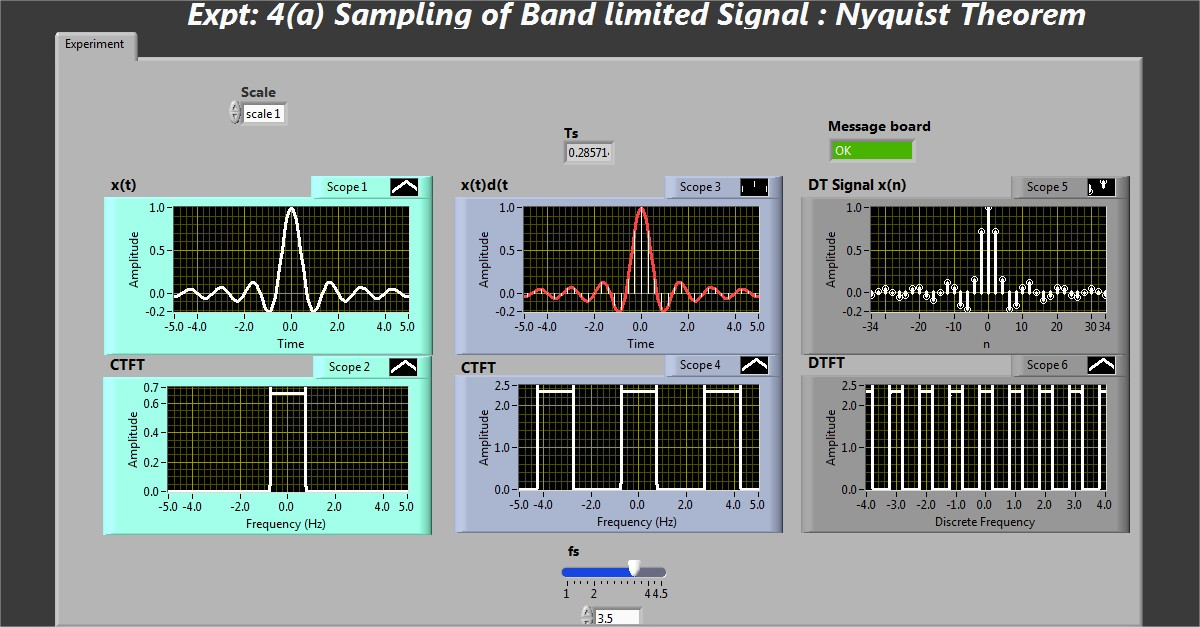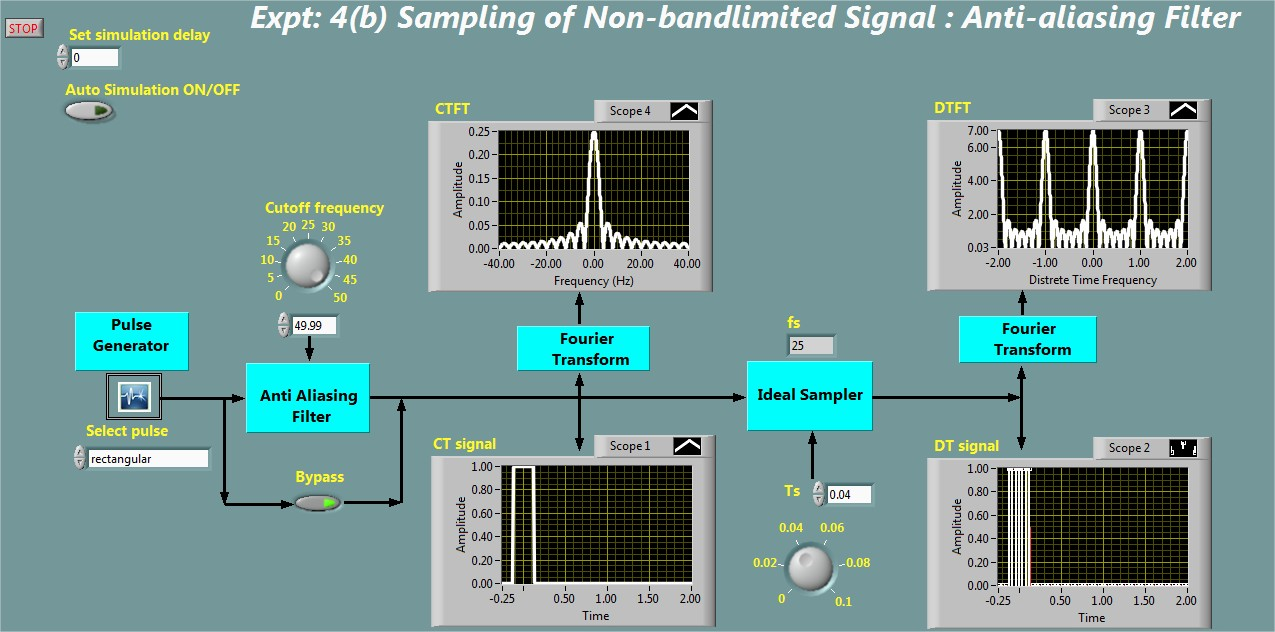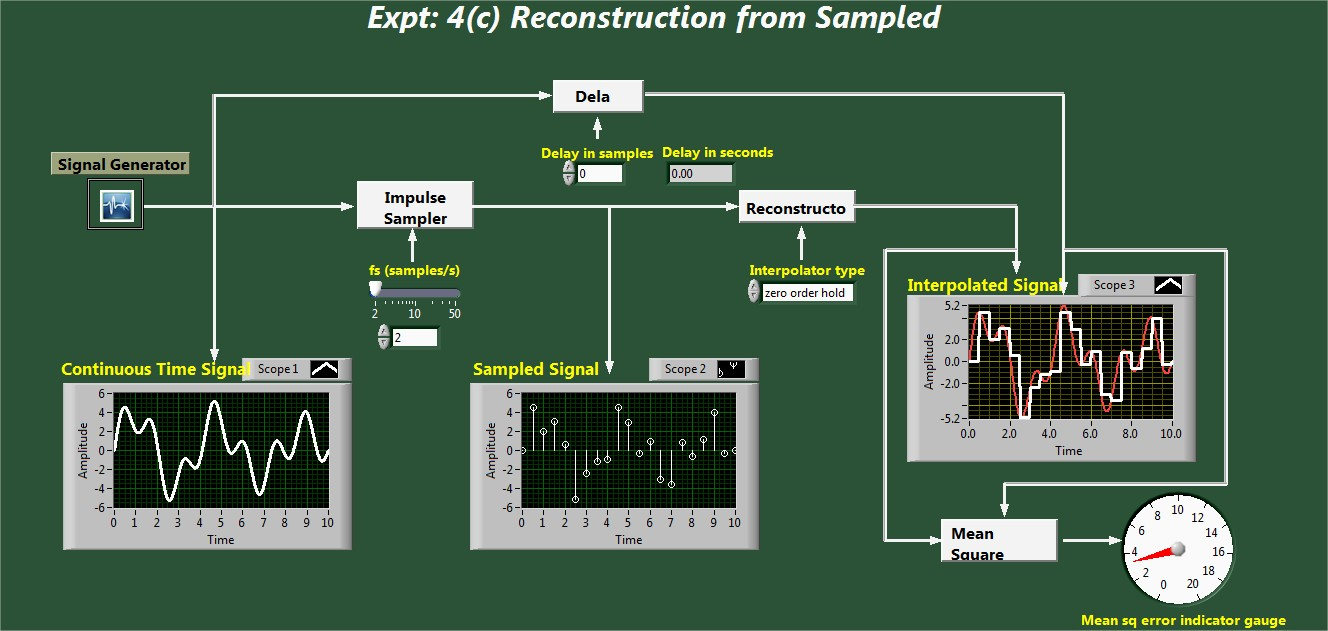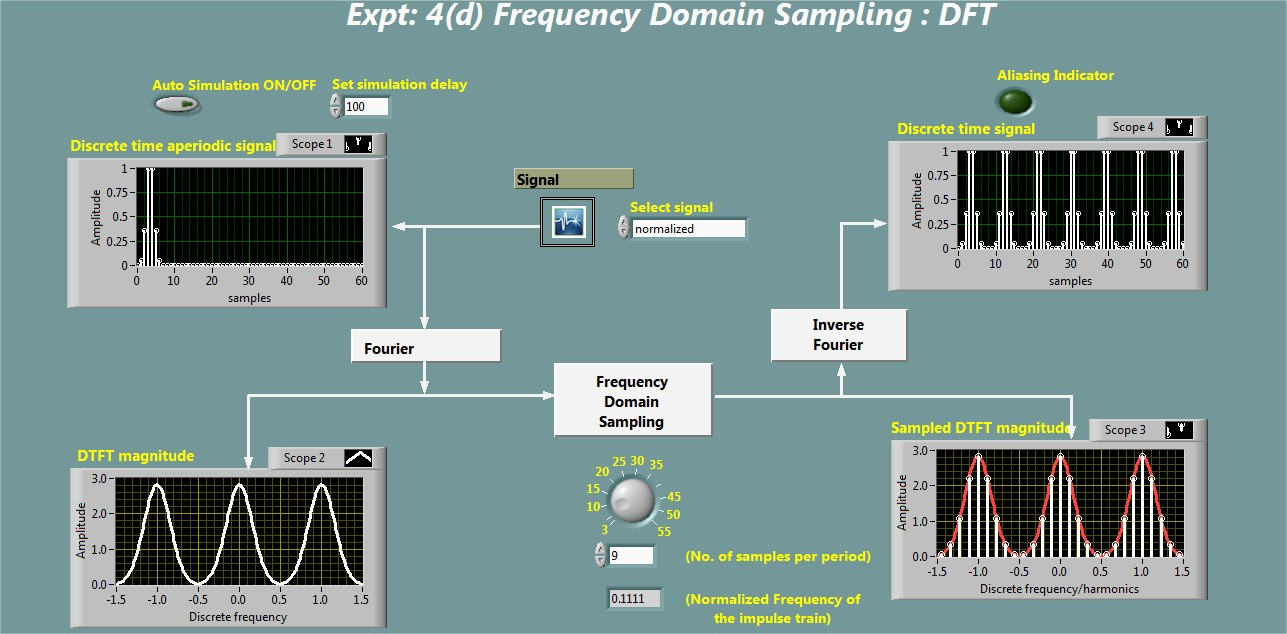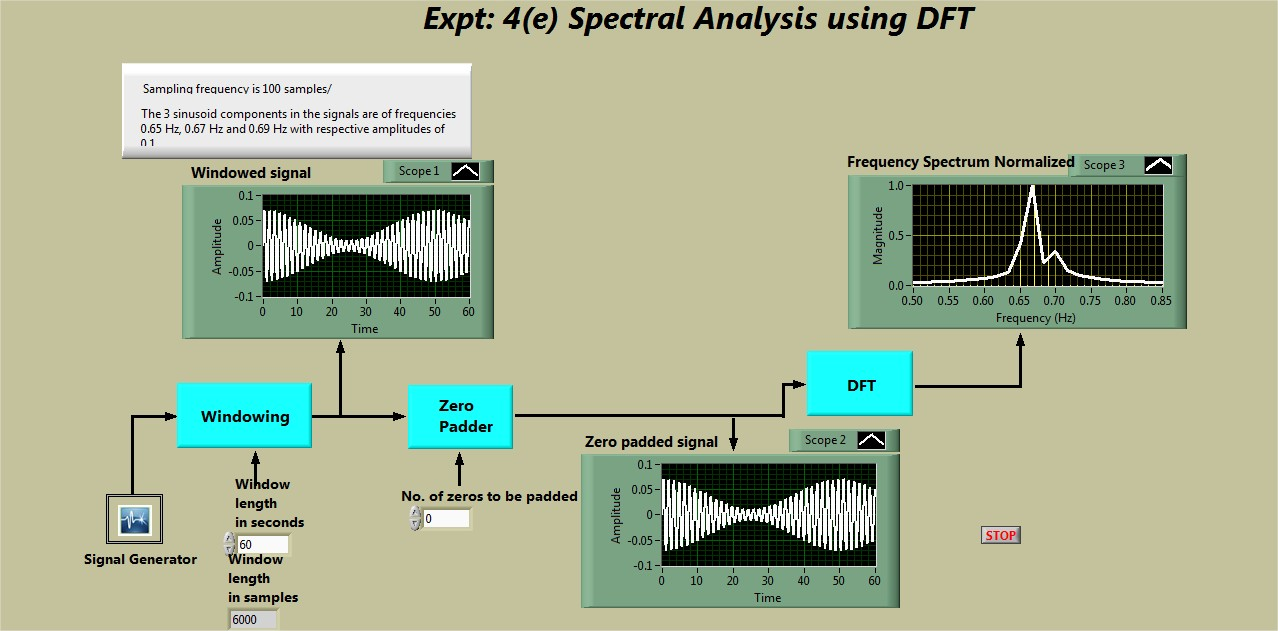# Sampling and signal Reconstruction

### Objectives

1. To demonstrate the time domain sampling of bandlimited signals (Nyquist theorem).

2. To demonstrate the time domain sampling of non-bandlimited signals and antialiasing filter.

3. To demonstrate the signal reconstruction using zero-order hold and first-order hold filters.

4. To demonstrate the sampling in frequency domain (Discrete Fourier Transform).

5. To demonstrate the spectral analysis using Discrete Fourier Transform.

Snapshot of the experiment windows